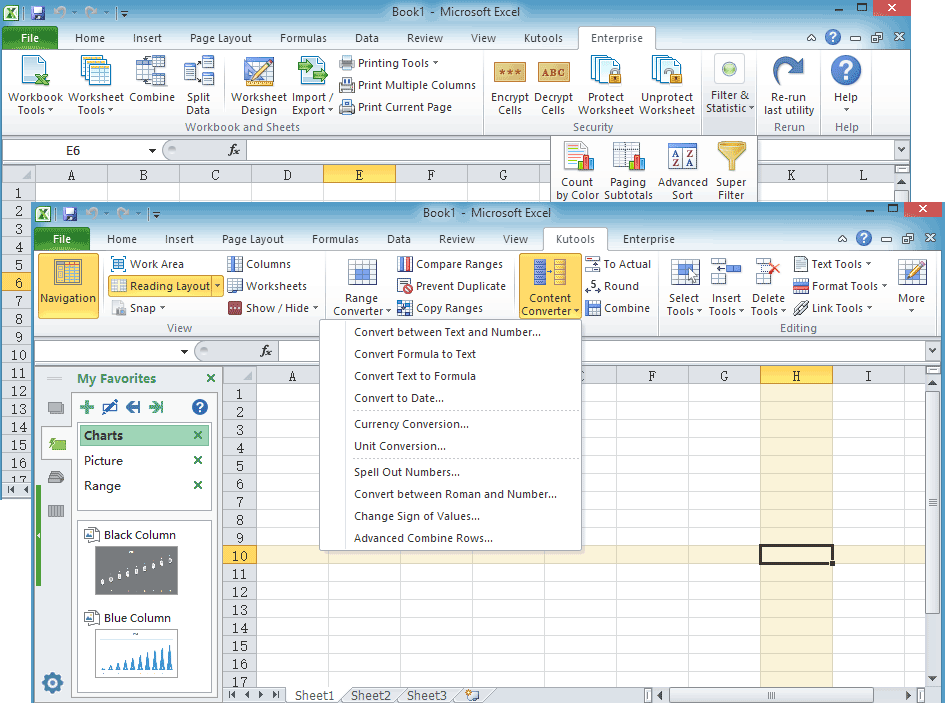Note: The other languages of the website are Google-translated. Back to English

## Excel calculate date and time: Add, subtract date time, time difference, age calculation

### Kutools for Excel

This tutorial introduces a powerful and helpful calculator which can solve almost 90% date and time calculations you probably use in Excel. It is the Date & Time Helper which is the one of features in Kutools for Excel.

What it can do are:

Add or Subtract date and time

Calculate the difference between two dates or two times and return a specified format

Calculate the Age based on a given date and return to year, month, day or year month day

#### Applying the Date & Time Helper feature by clicking Kutools > Formula Helper > Date & Time Helper.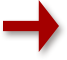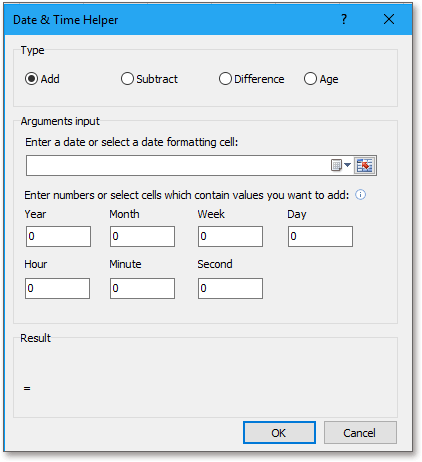#### Add or Subtract date and time

If you want to add or subtract year, month or days, or hours, minutes or seconds to a date time, you can use the first and second options in the Date & Time Helper dialog.

1. Select a cell which you want to place the calculation result at, then click Kutools > Formula Helper > Date & Time Helper to enable the Date & Time Helper dialog.

2. In the Date & Time Helper dialog, check Add or Subtract option in the Type section.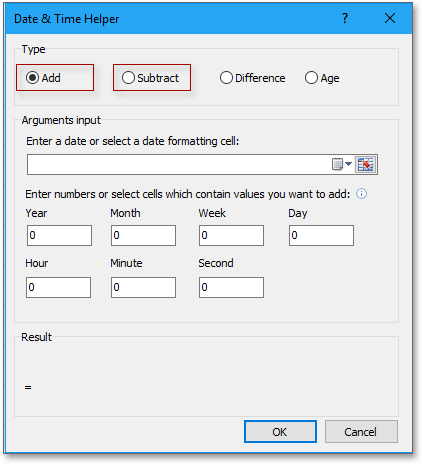3. Then in the Arguments input section, enter a date manually or click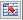to select a date to calculate in the text box of Enter a date or select a date formatting cell.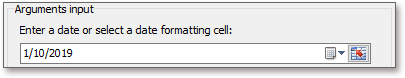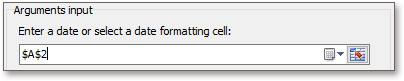4. Under Enter numbers or select cells which contain values you want to add (subtract), you can directly enter the numbers of year, month, week, day or hour, minute or seconds you want to add or subtract into the related textboxes, or you can select cells which contain the numbers you want to use to fill.

You can preview the formula and result in the Result section.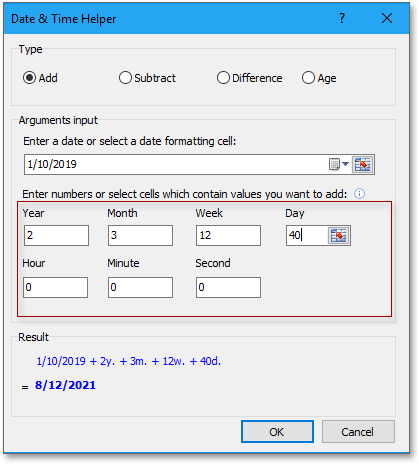5. Click OK. Then the calculated result has been displayed. And then you can drag the auto fill handle down to the cells for applying the formula.

Add Date (2 years, 3 months, 12 weeks and 40 days)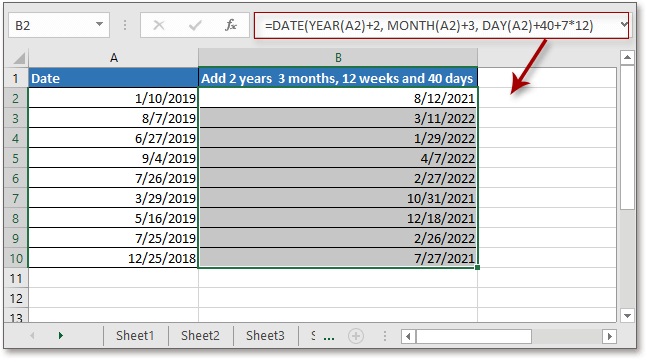Add date time (Add 3 days 3 hours 30 minutes and 15 seconds)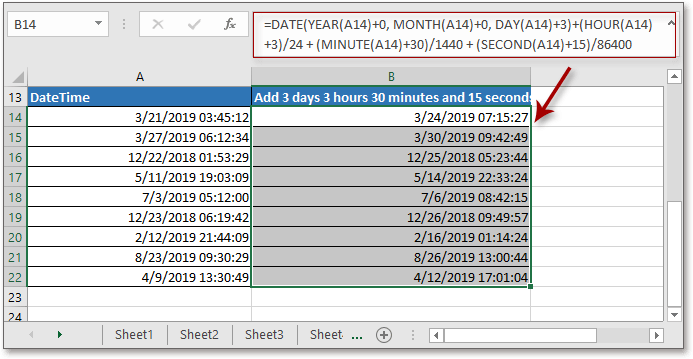Subtract datetime (4 weeks 1 hour)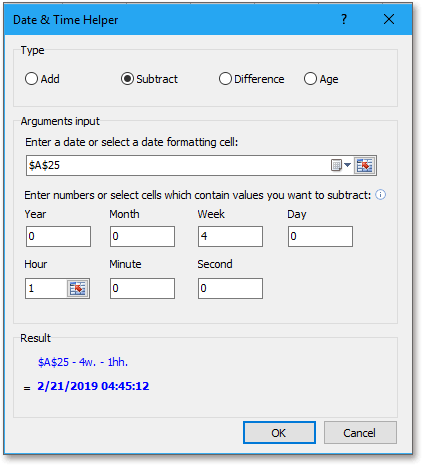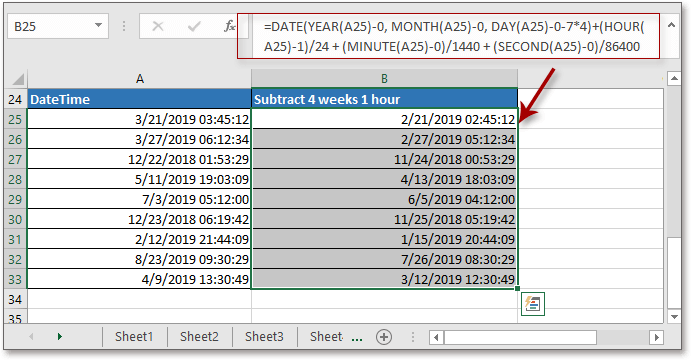#### Calculate the difference between two dates or two times and return a specified format

If you want to get the difference between two dates or two times, you can use the Difference option in the Date & Time Helper dialog.

1. Select a cell which you want to place the calculation result at, then click Kutools > Formula Helper > Date & Time Helper to enable the Date & Time Helper dialog.

2. In the Date & Time Helper dialog, check Difference option in the Type section.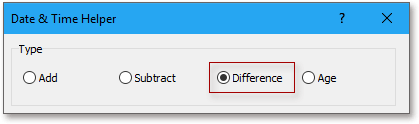3. Then in the Arguments input section, enter dates manually or clickto select dates to calculate in the text box of Date1 and Date2.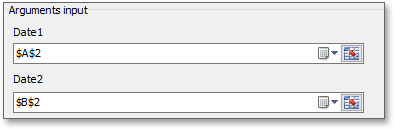4. Under Output result type, you can choose the format you want the result display as from the drop-down list.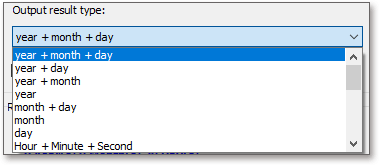You can preview the formula and calculation result in the Result section.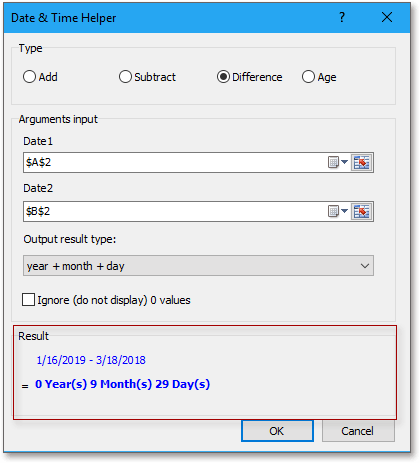Note: if you want to ignore the blank values, check Ignore (do not display) 0 values checkbox.

5. Click OK. Then the calculated result has been displayed. And then you can drag auto fill handle down to the cells for applying the formula.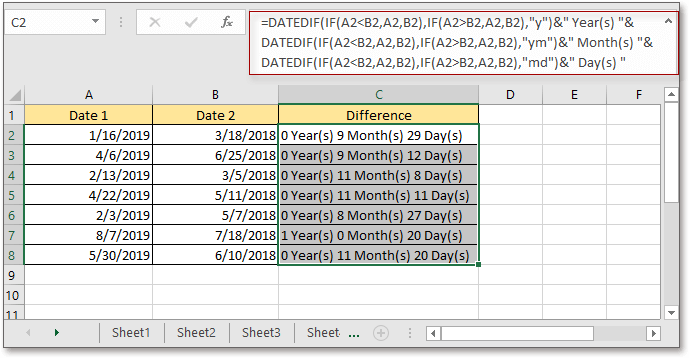#### Calculate the Age based on a given date and return to year, month, day or year month day

If you want to calculate the current age based on the birthday or a given date, you can choose the Age option.

1. Select a cell which you want to place the calculation result at, then click Kutools > Formula Helper > Date & Time Helper to enable the Date & Time Helper dialog.

2. In the Date & Time Helper dialog, check Age option in the Type section.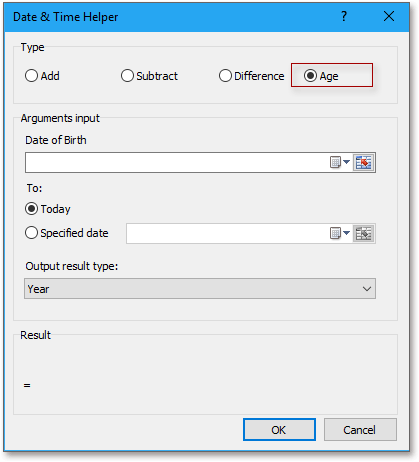3. Then in the Arguments input section, do as below:

1) Enter a date manually or clickto select a date to calculate in the text box of Date of Birth.

2) Check the end date as you need, if you want to calculate the current age, check Today, if you want to calculate the age on a specified date, check Specified date and then select the date cell.

3) Specify the result type as you need.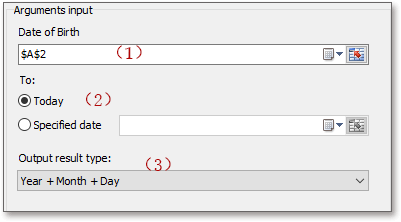You can preview the formula and calculation result in the Result section.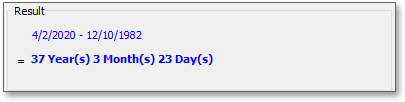4. Click OK. Then the calculated result has been displayed. And then you can drag the auto fill handle down to the cells for applying the formula.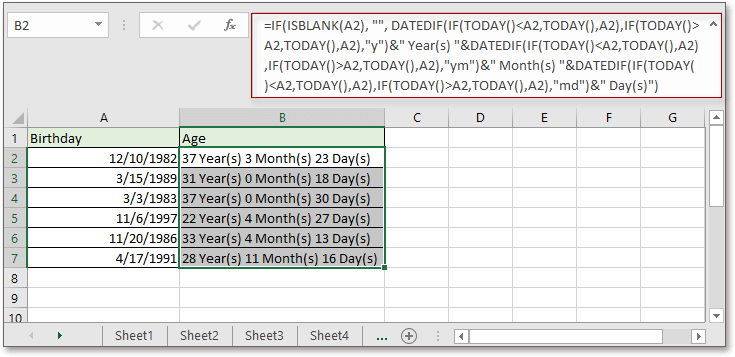Note：The Date & Time Helper supports Undo.

Productivity Tools Recommended
The following tools can greatly save your time and money, which one is right for you?
Office Tab: Using handy tabs in your Office, as the way of Chrome, Firefox and New Internet Explorer.
Kutools for Excel: More than 300 Advanced Functions for Excel 2021, 2019, 2016, 2013, 2010, 2007 and Office 365.

### Kutools for Excel

The functionality described above is just one of 300 powerful functions of Kutools for Excel.

Designed for Excel(Office) 2021, 2019, 2016, 2013, 2010, 2007 and Office 365. Free download and use for 60 days.